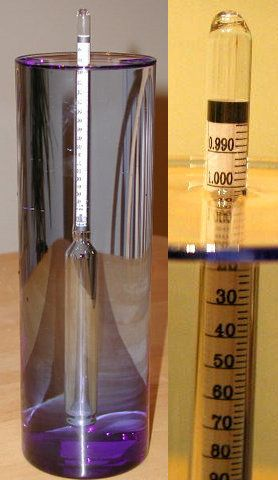# How Do Brewers Measure the Alcohol in Beer?A hydrometer used to measure the specific gravity of liquids. Notice the reading of 1.000 for water.

To determine the amount of alcohol in the beer they are making, brewers compare the specific gravity of the beer before it starts fermenting to its specific gravity when it is finished fermenting.

The specific gravity is a measure of the density of a liquid relative to water. The density of water is 1 kilogram per liter, so if the specific gravity of a liquid is 1.06, one liter of that liquid will weigh 1.06 kg.

The liquid that will form the beer is called a wort (pronounced wert). Its specific gravity is always higher than water because it contains a lot of dissolved sugars. Yeast added to the wort will convert some of these dissolved sugars into ethyl alcohol. When the beer is finished, the specific gravity is always less than when it started, because some of the sugars have been converted into alcohol, which is less dense than water (0.79 kg/L).

Glucose (C6H12O6) is the main sugar that will be converted to alcohol. Many reactions take place inside the yeast that ultimately convert each glucose molecule into two molecules of ethyl alcohol (CH3CH2OH) and two molecules of carbon dioxide (CO2).

C6H12O6 => 2(CH3CH2OH) + 2(CO2)

If you check the periodic table, you can figure out the molecular weights of these two molecules. The molecular weight of ethyl alcohol is 46.0688 and the molecular weight of carbon dioxide is 44.0098. You will need these numbers to calculate the alcohol content of the beer.

During the fermentation process most of the carbon dioxide that forms from the reaction bubbles out of the solution and leaves the fermentation vessel by way of a vent. You can say that all of it leaves, because the amount the remains in the beer is very small compared to the amount that leaves.

If you look at the equation for the reaction you see that each glucose is split into two ethyl alcohol molecules and two carbon dioxide molecules. That means for each carbon dioxide molecule that leaves the fermentation vessel, one ethyl alcohol molecule must be formed inside the vessel. If you look back at the molecular weights you can say that for each 44.0098 grams of CO2 that leaves the vessel 46.0688 grams of ethyl alcohol are formed. Put another way, for each gram of CO2 that bubbles off, about 1.05 grams of ethyl alcohol are produced.

You can compare the starting specific gravity to the final specific gravity. If the starting gravity of the wort is 1.06, and after fermentation, the gravity is 1.02. Subtracting the second from the first gives us the weight of CO2 that left the vessel. That is equal to 0.04 kg/L. Then you multiply by 1.05 to get the weight of the alcohol in the container. That is 0.042 kg/L. Now that you know both the mass of the solution (1.02 kg/L) and the mass of the alcohol (0.042 kg/L) you can calculate the percentage of alcohol by mass by dividing the two. This gives 0.042 / 1.02, which equals 0.041, or 4.1 percent.

It is important to note that the percentage of alcohol by mass is higher than the percentage of alcohol by volume because an equal mass of alcohol occupies more volume than water would. So to convert from percent alcohol by mass to percent alcohol by volume you just divide by the density of alcohol. In this case you get 4.1/0.79 or 5.2 percent alcohol by volume.

In Colorado the beer sold in grocery stores has to be low alcohol "3.2" beer. It can contain no more than 3.2 percent alcohol by mass. But the beer sold in liquor stores is labeled by volume, and most beer is about 5 percent alcohol by volume. But when you convert from weight to volume you find that the "3.2" beer is really 4 percent alcohol by volume. Still a pretty big difference, but maybe not quite as big as you might think.